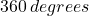# C Program To Identify A Quadrilateral

The program to identify a quadrilateral read inputs – length of sides and angles made by the quadrilateral shape and prints the shape name as output.

We compiled the program using Dev-C++ compiler installed on a windows 7 64-bit system. You may try another standard C compiler, but make sure to modify the code according to your compiler specifications.

You must be aware of following C programming concept to understand this example program.

Before you practice this program, you must go through the concepts of quadrilateral shapes and their differences. See the problem definition section.

## Problem Definition

A quadrilateral is a polygon shape that has 4 sides and corners that make 4 angles. The sum of those angles must be equal to. There are many types of quadrilateral shapes, you will find a brief description of each shape below.

### Square

A square has 4 equal sides and 4 right angles at each corner.

### Rectangle

A rectangle has 4 sides with parallel sides are of the same length and 4 corners are right angles. The rectangle has a longer side and a shorter side.

### Rhombus

A rhombus is like a square or diamond with 4 equal sides, but none of the corners is a right angle.

### Parallelogram

Any quadrilateral that has a pair of parallel sides is a parallelogram. There are shapes which demonstrate properties of parallelogram – square, rectangle, and a rhombus are special case of a parallelogram.

### Trapezoid

If a quadrilateral contains at least one pair of parallel lines then it is called a trapezoid.

### Kite

A kite is a quadrilateral that has a pair of adjacent sides of equal length and the angle where these two pair meet are equal.

## Program Code

#include <stdio.h>
#include <stdlib.h>
int main()
{
float a, b, c, d, sum = 0.0;
float angle, arr, len, tmp; int i;
int flag, square, rectangle, rhombus, para, trap;
flag = square = rectangle = rhombus = trap = 0;
para = 0;
printf("Enter length of all sides of the Quadrilateral:");
scanf("%f", & arr);
scanf("%f", & arr);
scanf("%f", & arr);
scanf("%f", & arr);
len = arr;
printf("Enter Angles of all Corners of the Quadrilateral:");
for (i = 0; i < 4; i++)
{
scanf("%f", & angle[i]);
if (angle[i] == 90)
{
flag++;
}
}

/* 360 degree check */
for (i = 0; i < 4; i++)
{
sum = sum + angle[i];
}
if (sum == 360)
{
printf("sum of angle is 360 n");
}
else
{
printf("Sum of Angles is not 360:n");
abort();
}

/* Identify Square */
for (i = 0; i < 4; i++)
{
if (arr[i] == len)
{
square++;

if (square == 4 && flag == 4)
{
for (i = 0; i < 30; i++)
printf("_"); printf("\n\n");
printf("It is a Square:\n\n");
for (i = 0; i < 30; i++)
printf("_"); printf("\n\n");
}
}
}

/* Identify rectangle */
if (arr == arr && arr == arr && arr != arr && flag == 4)
{
for (i = 0; i < 30; i++)
printf("_"); printf("\n\n");
printf("It is a Rectangle:\n\n");
for (i = 0; i < 30; i++)
printf("_"); printf("\n\n");
}

/* identify parallelogram */
for (i = 0; i < 4; i++)
{
if (angle[i] == 90)
{
para++;
}
}
if (arr == arr || arr == arr)
{
if (para < 4)
{
for (i = 0; i < 30; i++)
printf("_"); printf("\n\n");
printf("It is a Parallelogram :\n\n");
for (i = 0; i < 30; i++)
printf("_"); printf("\n\n");
}
}

/* Identify Kites */
if (arr == arr || arr == arr)
{
if (para < 3 && angle == angle && angle == angle)
{
if (arr != arr)
for (i = 0; i < 30; i++)
printf("_"); printf("\n\n");
printf("It is a Kite :\n\n");
for (i = 0; i < 30; i++)
printf("_"); printf("\n\n");
}
}

/* Identify rhombus */
for (i = 0; i < 4; i++)
{
if (arr[i] == len)
{
rhombus++;
if (rhombus == 4 && angle[i] != 90)
{
for (i = 0; i < 30; i++)
printf("_"); printf("\n\n");
printf("It is a Rhombus:\n\n");
for (i = 0; i < 30; i++)
printf("_"); printf("\n\n");
}
}
}

/* Identify trapezoid */
for (i = 0; i < 4; i++)
{
len = arr[i];
tmp = angle[i];
for (i = 0; i < 4; i++)
{
if (arr[i + 1] != len && angle[i] != tmp)
{
trap++;
}
}
}

if (trap == 3)
{
for (i = 0; i < 30; i++)
printf("_"); printf("\n\n");
printf("It is a Trapezoid!\n");
for (i = 0; i < 30; i++)
printf("_"); printf("\n\n");
}
system("PAUSE");
return 0;
}

## Output

Enter length of all sides of the Quadrilateral:20
20
20
20
Enter Angles of all Corners of the Quadrilateral:90
90
90
90
sum of angle is 360
________________________________
It is a Square:
________________________________
Enter length of all sides of the Quadrilateral:12
12
12
12
Enter Angles of all Corners of the Quadrilateral:60
120
60
120
sum of angle is 360
____________________________
It is a Parallelogram :
____________________________
____________________________
It is a Rhombus:
____________________________